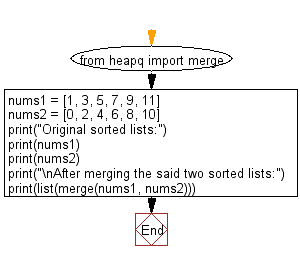﻿ Python: Combine two given sorted lists using heapq module - w3resource# Python: Combine two given sorted lists using heapq module

## Python List: Exercise - 152 with Solution

Write a Python program to combine two given sorted lists using heapq module.

Sample Solution:

Python Code:

``````from heapq import merge
nums1 = [1, 3, 5, 7, 9, 11]
nums2 = [0, 2, 4, 6, 8, 10]
print("Original sorted lists:")
print(nums1)
print(nums2)
print("\nAfter merging the said two sorted lists:")
print(list(merge(nums1, nums2)))
```
```

Sample Output:

```Original sorted lists:
[1, 3, 5, 7, 9, 11]
[0, 2, 4, 6, 8, 10]

After merging the said two sorted lists:
[0, 1, 2, 3, 4, 5, 6, 7, 8, 9, 10, 11]
```

Flowchart:## Visualize Python code execution:

The following tool visualize what the computer is doing step-by-step as it executes the said program:

Python Code Editor:

Have another way to solve this solution? Contribute your code (and comments) through Disqus.

What is the difficulty level of this exercise?

Test your Python skills with w3resource's quiz

﻿

## Python: Tips of the Day

Floor Division:

When we speak of division we normally mean (/) float division operator, this will give a precise result in float format with decimals.

For a rounded integer result there is (//) floor division operator in Python. Floor division will only give integer results that are round numbers.

```print(1000 // 300)
print(1000 / 300)```

Output:

```3
3.3333333333333335```